Tetrapack

How high should be the milk box in the shape of a prism with base dimensions 8 cm and 8.8 cm if its volume is 1 liter?

Result

x =  14.2 cm

Solution:Leave us a comment of example and its solution (i.e. if it is still somewhat unclear...):Be the first to comment!To solve this verbal math problem are needed these knowledge from mathematics:

Do you want to convert length units? Do you know the volume and unit volume, and want to convert volume units?

Next similar examples:

1. Truck of milk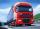How many hectoliters of ,,the box" milk fit in the truck, ake cargo size area is 2.8 m x 3 m x 17 m? A liter of milk in a box measuring 12 cm x 7 cm x 20 cm.
2. Concrete pillar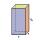How many m³ of concrete is needed for the construction of the pillar shape of a regular tetrahedral prism, when a = 60 cm and the height of the pillar is 2 meters?
3. Rectangular prismIf i have a rectangular prism with a length of 1,000 cm, width of 30 cm and a height of 50 cm, what is the volume?
4. Cubes into cuboidHow many 12 centimeter cubes fit into the block (cuboid) with 6dm, 8,4dm and 4,8?
5. The shopThe shop has 3 hectoliters of water. How many liter bottles is it?
6. Clay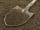How many cubic centimeters of clay is in a pit of dimensions 4 m x 3 m x 3 m?
7. The wall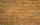We have to build a cuboid wall with dimensions base 30 cm and 45 cm and height 3.25 meters. Calculate how many we need bricks if we spend 400 pieces of bricks to 1 m3 of wall?
8. AquariumAquarium is rectangular box with square base containing 76 liters of water. Length of base edge is 42 cm. To what height the water level goes?
9. Cylindrical tank 2If a cylindrical tank with volume is used 12320cm raised to the power of 3 and base 28cm is used to store water. How many liters of water can it hold?
10. HectolitersHow many hectoliters of water fits into cuboid tank with dimensions of a = 3.5 m b = 2.5 m c = 1.4 m?
11. Fire tankHow deep is the fire tank with the dimensions of the bottom 7m and 12m, when filled with 420 m3 of water?
12. Water reservoirThe water tank has a cuboid with edges a= 1 m, b=2 m , c = 1 m. Calculate how many centimeters of water level falls, if we fill fifteen 12 liters cans.
13. Caleb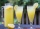Caleb is making lemonade for a party. He has 5 gallons of lemonade. He is putting 1/3 cubic inch of lemonade in a cup for each guest. How many guests are going to be at the party?
14. Customary lengthConvert length 65yd 2 ft to ft
15. Jill's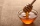Jill's mom stored some of the honey in a container that held 3/4 of a gallon. She used half of this amount to sweeten tea. How much honey, in cups was used in the tea. Express your answers in cups.
16. Two cuboidsFind the volume of cuboidal box whose one edge is: a) 1.4m and b) 2.1dm
17. StepsWalkway from the house to the school is 180 m long. How many more steps Michael makes than his father on the way to school, if the length of Michael's step is 60 cm and the length of his father's step is 90 cm.